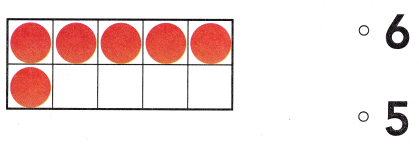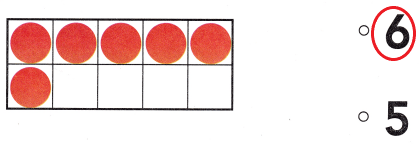# Texas Go Math Kindergarten Lesson 4.1 Answer Key Model and Count 6

Refer to our Texas Go Math Kindergarten Answer Key Pdf to score good marks in the exams. Test yourself by practicing the problems from Texas Go Math Kindergarten Lesson 4.1 Answer Key Model and Count 6.

## Texas Go Math Kindergarten Lesson 4.1 Answer Key Model and Count 6

Explore

DIRECTIONS: Place a counter on each ticket in the set as you count them. Move the counters to the ten frame. Draw the counters.Explanation:
I placed counters on each ticket and moved them to the ten-frame.There are 6 tickets.So, i drew 6 counters.

Share and Show

DIRECTIONS: 1. Place a counter on each car in the set as you count them. Move the counters to the parking lot. Draw the counters.

Question 1.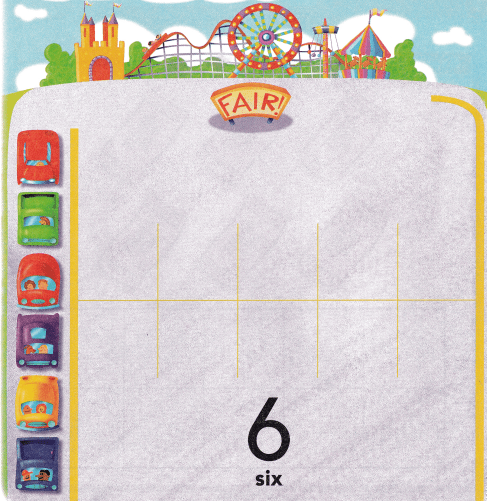Explanation:
I placed counters on each car and moved them to the ten-frame.There are 6 tickets.So, i drew 6 counters.

DIRECTIONS: 2. Place two-color counters in the ten frame to model the different ways to make 6. Write to show some pairs of numbers that make 6.

Question 2.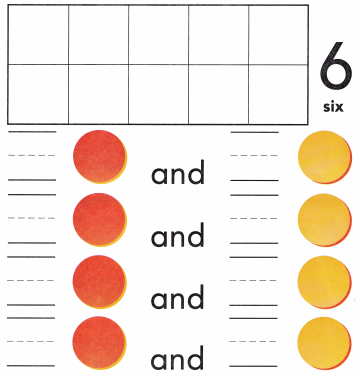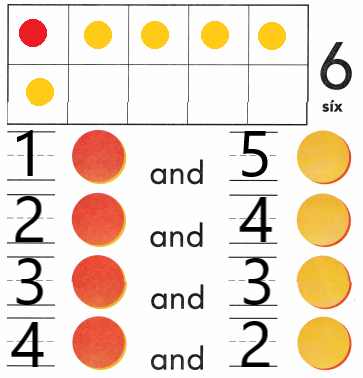Explanation:
I placed two-color counters 1 red counter and 5 yellow counters in the ten frame to model the different ways to make 6 and write to show some pairs of numbers that make 6.They are, 1 and 5, 2 and 4, 3 and 3 and 4 and 2.

HOME ACTIVITY • Ask your child to show a set of five objects. Have him or her show one more object and tell how many objects are in the set.

DIRECTIONS: 3. Six people bought popcorn. Count the buckets of popcorn in each set. Circle the sets that show six buckets. 4. Choose the correct answer. What number does the model show?

Problem Solving

Question 3.Explanation:
There are 6 counters in the above ten-frame.So, i counted and marked the number 6.

Question 4.Explanation:
There are 6 counters in the above ten-frame.So, i counted and marked the number 6.

### Texas Go Math Kindergarten Lesson 4.1 Homework and Practice Answer Key

DIRECTIONS: 1. Mark an X on each rocket ship as you count them. Draw a counter on the ten frame as you count each rocket ship.

Question 1.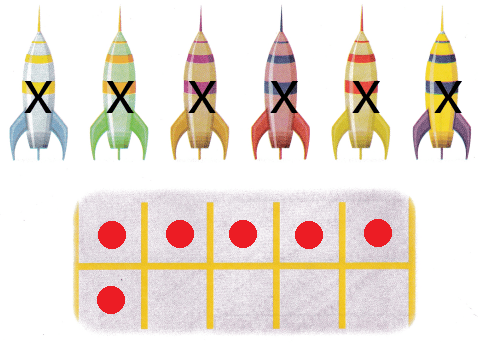Explanation:
There are 6 rockets in the image.So, i counted and drew as many counters as the number 6 in the above ten-frame.

DIRECTIONS: Choose the correct answer. 2-4. What number does the model show?

Lesson Check

Question 2.Explanation:
There are 5 counters in the above ten-frame.So, i counted and marked the number 5.

Question 3.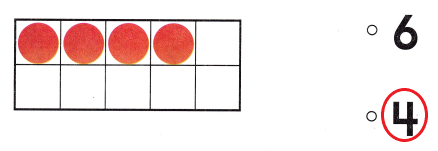Explanation:
There are 6 counters in the above ten-frame.So, i counted and marked the number 6.

Question 4.